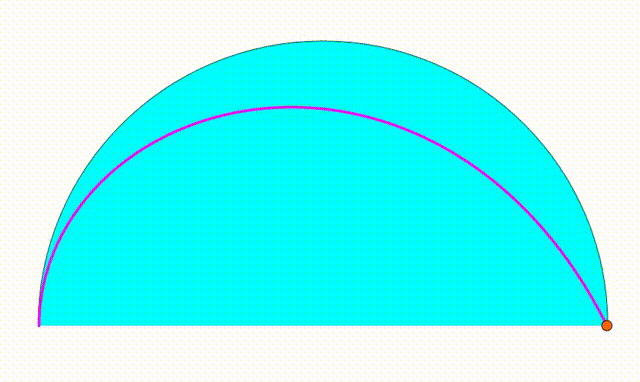# Dynamic Geometry: P77

Geometry Level pendingThe diagram shows a black semicircle with radius $1$. The cyan and green semicircles are tangent to each other and internally tangent to the black semicircle. They are growing and shrinking freely so that the sum of their radius is always equal to $1$. We draw a red vertical segment using their tangency point. At last we inscribed two yellow circles so they are tangent to the red line, to the black semicircle and to one of the two bottom semicircles. The center of one yellow circle (orange point) traces a locus (purple curve). The area bounded by the purple curve and the black semicirle's diameter can be expressed as $\dfrac{p}{q}$, where $p$ and $q$ are coprime positive integers. Find $p-q$.

×